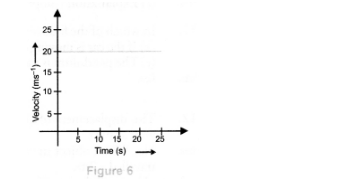# The velocity-time graph (Fig. 6 ) shows the motion of a cyclist.`
Question:

The velocity-time graph (Fig. 6 ) shows the motion of a cyclist. Find

(i) its acceleration

(ii) its velocity and

(iii) the distance covered by the cyclist in 15 seconds.Solution:

(i) Acceleration $=\frac{\text { Change in velocity }}{\text { time }}=0$

(ii) Velocity $=20 \mathrm{~m} \mathrm{~s}^{-1}$

(iii) Distance $=$ area under $v-t$ graph

$=v \times t=20 \mathrm{~ms}^{-1} \times 15 \mathrm{~s}=300 \mathrm{~m} .$